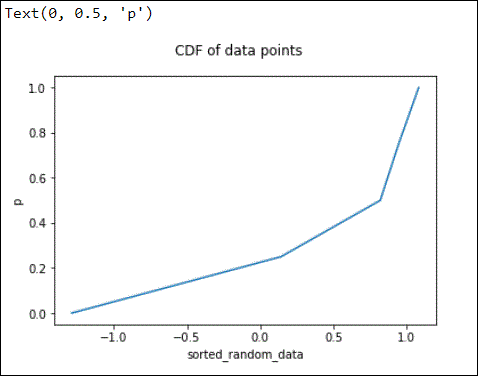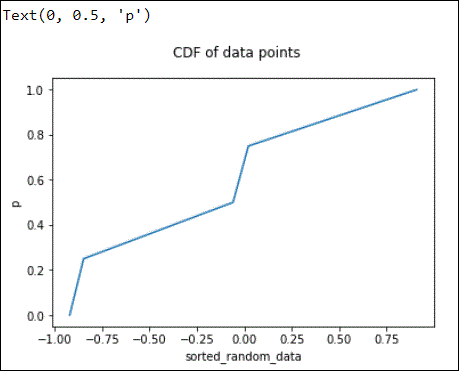# Calculate the Cumulative Distribution Function in Python

Najwa Riyaz Oct 12, 2021 Jun 01, 2020 Python Python Math

The term cumulative distribution function or `CDF` is a function `y=f(x)`, where `y` represents the probability of the integer `x`, or any number lower than `x`, being randomly selected from a distribution.

It is calculated in Python by using the following functions from the `NumPy` library.

1. `numpy.arange()` function which returns an `ndarray` of evenly spaced values.
2. `numpy.linspace()` function which returns an `ndarray` of evenly spaced values within a given interval.

## Use `numpy.arange()` to Calculate the CDF in Python

The `NumPy` standard library contains the `arange()` function used to determine the CDF in Python.

For this, import the `NumPy` library first.

The `arange()` function returns an `ndarray` of evenly spaced values.

Below is an example that demonstrates the implementation of the CDF function using the `numpy.arange()` function in Python.

``````import matplotlib.pyplot as plt
import numpy

data = numpy.random.randn(5)
print("The data is-",data)
sorted_random_data = numpy.sort(data)
p = 1. * numpy.arange(len(sorted_random_data)) / float(len(sorted_random_data) - 1)
print("The CDF result is-",p)

fig = plt.figure()
fig.suptitle('CDF of data points')
ax2.plot(sorted_random_data, p)
ax2.set_xlabel('sorted_random_data')
ax2.set_ylabel('p')
``````

Here, the `randn()` function is used to return samples of data using the standard normal distribution. Since `randn(5)` is mentioned, a 1Darray is built with 5 random values.

Next, the data is sorted using the `sort()` function, after which the `arange()` function is used to calculate the CDF.

Output:

``````The data is- [ 0.14213322 -1.28760908  0.94533922  0.82004319  1.08232731]
The CDF result is- [0.   0.25 0.5  0.75 1.  ]
``````

The graph is displayed as per the CDF function as.## Use `numpy.linspace()` to Calculate the CDF in Python

The `NumPy` standard library contains the `linspace()` function used to determine the CDF in Python. For this, import the `NumPy` library first.

The `linspace()` function returns an `ndarray` of evenly spaced numbers over a specified interval.

Here is an example that demonstrates the implementation of the CDF function using `numpy.linspace()` in Python.

``````import matplotlib.pyplot as plt
import numpy as np

data = np.random.randn(5)
print("The data is-",data)
sorted_random_data = np.sort(data)
np.linspace(0, 1, len(data), endpoint=False)

print("The CDF result using linspace =\n",p)

fig = plt.figure()
fig.suptitle('CDF of data points')
ax2.plot(sorted_random_data, p)
ax2.set_xlabel('sorted_random_data')
ax2.set_ylabel('p')
``````

Here, the `randn()` function is used to return samples of data using the standard normal distribution. Next, the data is sorted using the `sort()` function, after which the `arange()` function is used to calculate the CDF.

Output:

``````The data is- [-0.92106668 -0.05998132  0.02102705 -0.84778184  0.90815869]
The CDF result using linspace =
[0.   0.25 0.5  0.75 1.  ]
``````

The graph is displayed as per the CDF function as below.## Related Article - Python Math

• Calculate Factorial in Python
• Calculate Inverse of Cosine in Python
• Calculate Modular Multiplicative Inverse in Python
• Fit Poisson Distribution to Different Datasets in Python
• Reduce Fractions in Python
• Define an Infinite Value in Python